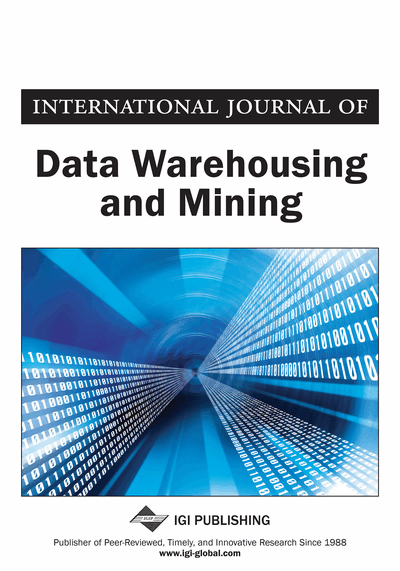# A Mathematical Database to Process Time Series

Cyrille Ponchateau, Ladjel Bellatreche, Carlos Ordonez, Mickael Baron
DOI: 10.4018/IJDWM.2018070101
OnDemand:
(Individual Articles)
Available
\$37.50
No Current Special Offers

## Abstract

In scientific research, the results of an experiment commonly take the form of a time series, in which such time series consists of measurements collected from a sensor over time. After time series are stored, mathematical models are derived using numerical methods. Even though there exist plenty of tools to store and analyze time series data, there is scarce research aimed at storing and querying derived models, which are the most important mechanism for a scientist to understand data. In this article, the authors propose to help scientists with a flexible database structure to persist and manage mathematical models with a mathematical models store, with extended features, to handle time series. In this article, the authors introduce the concept of a mathematical models store enriched with numerical processing methods to allow queries based on raw time series data. Then they introduce a prototype, that is an implementation of such a data store with PostgreSQL.
Article Preview
Top

## Introduction

The evolution of computer technology in terms of computing and storage capacity, now allows the processing of large data sets containing complex numerical data, a typical task for mathematical software. Such data sets are commonly found in experimental sciences (Shatkay & Zdonik, 1996), from medicine (medical imaging (Bagnall, Ratanamahatana, Keogh, Lonardi, & Janacek, 2006), electrocardiogram (Lang, Morse, & Patel, 2010; Fu, 2011) and medical surveillance (Esling & Agón, 2012)) to physics (particle tracking (Lang et al., 2010)). But also in other domains such as finance (weekly sales total (Fu, 2011), stock price movements (Lee, Kwon, & Lee, 2003)).

This work, in particular, was initiated by an automatic control team. In their domain (as in other experimental sciences domains), the researchers design experiments to study physical systems. For instance, when a voltage is applied at the terminals of an electrical motor, it starts rotating and the rotating speed depends on the aforementioned voltage. Then, the aim of the automatic control researcher is to mathematically describe the dependence between voltage and rotating speed. Thereafter, the motor is tested with different voltages and the rotating speed evolution is measured by a sensor. The measurements are taken at a fixed rate, generating a series of chronological values, represented as a time series. The next step consists in analyzing the time series, using numerical software such as Matlab, Octave, R, and so on. Finally, the analysis provides a mathematical model (a differential equation), describing how the motor rotating speed behaves according to the voltage variations.

From a practical standpoint, when models are produced, in general, researchers have no standard structure or format to store them. Therefore, they end up being stored in different formats in many files in a disorganized manner. For instance, models are sometimes embedded in Matlab, R or Python scripts. Moreover, they can be stored on text files, spreadsheets, word processing files and so on. As a result, finding a particular model requires a long and cumbersome search among different files and directories. In order to develop a solution that provides both standardization and organization to avoid manual search and retrieval of models, the authors propose a system with two major features: (1) storing model equations and numeric data in a database, (2) adding a numerical processing software layer connected to the DBMS.

Currently, there exist mature technologies that allow storage of massive time series (TokuDB (Namiot, 2015; Bartholomew, 2014), Vertica (Lamb et al., 2012), OpenTSDB (Dunning & Friedman, 2015), etc). However, just storing the time series is not enough to satisfy analysts’ needs. Indeed, the goal of experimental scientists is the derivation of mathematical models computed to describe in an abstract manner the observations they make (i.e. fitting a model to the time series data). This fact is especially important when the aforementioned model is not a simple mathematical formula, but a concrete and detailed set of mathematical expressions explaining how the observed system behaves and why. The authors defend the idea that such information is more crucial to persist than the observed time series itself. Also, since mathematics provides general tools to solve diverse problems, one model can be applied on different systems. Keeping this motivation in mind, the authors’ goal is to provide a standard solution to represent, store and exchange models, and take profit of this organized storage of models to help the user retrieve a model based on his raw experimental numeric data.

## Complete Article List

Search this Journal:
Reset
Volume 19: 6 Issues (2023)
Volume 18: 4 Issues (2022): 2 Released, 2 Forthcoming
Volume 17: 4 Issues (2021)
Volume 16: 4 Issues (2020)
Volume 15: 4 Issues (2019)
Volume 14: 4 Issues (2018)
Volume 13: 4 Issues (2017)
Volume 12: 4 Issues (2016)
Volume 11: 4 Issues (2015)
Volume 10: 4 Issues (2014)
Volume 9: 4 Issues (2013)
Volume 8: 4 Issues (2012)
Volume 7: 4 Issues (2011)
Volume 6: 4 Issues (2010)
Volume 5: 4 Issues (2009)
Volume 4: 4 Issues (2008)
Volume 3: 4 Issues (2007)
Volume 2: 4 Issues (2006)
Volume 1: 4 Issues (2005)
View Complete Journal Contents Listing